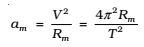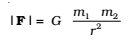Gravitation is the name given to the force of attraction acting between any two bodies of the universe.

Newton’s reasoning was that the moon revolving in an orbit of radius Rm was subject to a centripetal acceleration due to earth’s gravity of magnitudewhere V is the speed of the moon related to the time period T by the relation V = 2π Rm /T . The time period T is about 27.3 days and Rm was already known then to be about 30.84 × 108m.

If we substitute these numbers in above equation, we get a value of am much smaller than the value of acceleration due to gravity g on the surface of the earth, arising also due to earth’s gravitational attraction.

## Universal Law of Gravitation:

Everybody in the universe attracts every other body with a force which is directly proportional to the product of their masses and inversely proportional to the square of the distance between them.where G is the universal gravitational constant.

### Gravitational constant (G):

It is equal to the force of attraction acting between two bodies each of unit mass, whose centers are placed unit distance apart. Value of G is constant throughout the universe. It is a scalar quantity. The dimensional formula G = [M-1 L3 T-2]. In SI unit, the value of

G =6.67X10-11 Nm2 kg-2.